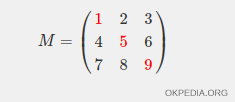# How to replace diagonals of a matrix in Matlab and Octave

To replace the diagonal elements in a matrix use the function spdiags()

spdiags(v,i,m)

• The first parameter is an array with the new diagonal elements.
• The second parameter is the diagonal index (0 is the main diagonal)
• The third parameter is the name of the array.

This function modifies the values of the i-th diagonal of the matrix.

What are the diagonals of a matrix? The main diagonal of the matrix starts at the top right and ends at the bottom left or vice versa. For example, the main diagonal of the matrix M are the elements 1, 5, 9.## Examples

Example 1

Create a matrix

>> M=[1 2 3 ; 4 5 6 ; 7 8 9]
M =
1 2 3
4 5 6
7 8 9

To replace the elements on the main diagonal use the function spdiags()

>> spdiags([-1;-2;-3],0,M)

The output result is a new matrix with the new elements -1, -2, -3 on the main diagonal

-1 2 3
4 -2 6
7 8 -3

Example 2

To replace the elements above the main diagonal, type

>> spdiags([-1;-2],1,M)

The output result is a new matrix with the new elements -1, -2 on the diagonal above the main diagonal

1 -1 3
4 5 -2
7 8 9

https://how.okpedia.org/en/matlab/how-to-replace-diagonals-of-a-matrix-in-matlab-and-octaveReport us an error or send a suggestion to improve this page

Matrix in Matlab/Octave